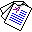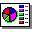# Linear Algebraic Characterization of Logic Programs

## Chiaki Sakama, Katsumi Inoue and Taisuke Sato

in: Proceedings of the 10th International Conference on Knowledge Science, Engineering and Management (KSEM 2017)

## Abstract

This paper introduces a novel approach for computing logic programming semantics based on multilinear algebra. First, a propositional Herbrand base is represented in a vector space and if-then rules in a program are encoded in a matrix. Then we provide methods of computing the least model of a Horn logic program, minimal models of a disjunctive logic program, and stable models of a normal logic program by algebraic manipulation of higher-order tensors. The result of this paper exploits a new connection between linear algebraic computation and symbolic computation, which has potential to realize logical inference in huge scale of knowledge bases.Full Paper (PDF 146K)Slide